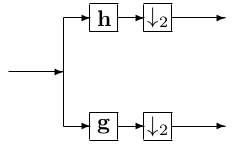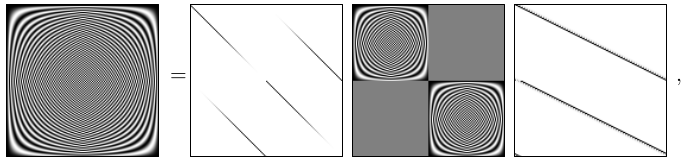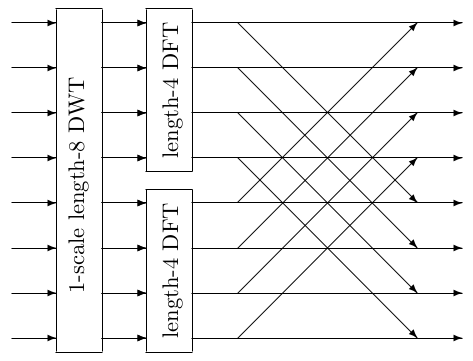0.10 Wavelet-based signal processing and applications  (Page 4/13)

 Page 4 / 13Building Block for the Discrete Wavelet Transform

The computational complexity of the DWT algorithm can also be easily established. Let ${C}_{DWT}\left(N\right)$ be the complexity for a length-N DWT. Since after each scale, we only further operate on half of the output data, wecan show

${C}_{DWT}\left(N\right)=O\left(N\right)+{C}_{DWT}\left(N/2\right),$

which gives rise to the solution

${C}_{DWT}\left(N\right)=O\left(N\right).$

The operation in [link] can also be expressed in matrix form ${\mathbf{W}}_{N};$ e.g., for Haar wavelet,

${\mathbf{W}}_{4}^{Haar}=\sqrt{2}/2\left[\begin{array}{cccc}\hfill 1& \hfill -1& \hfill 0& \hfill 0\\ \hfill 0& \hfill 0& \hfill 1& \hfill -1\\ \hfill 1& \hfill 1& \hfill 0& \hfill 0\\ \hfill 0& \hfill 0& \hfill 1& \hfill 1\end{array}\right].$

The orthogonality conditions on $\mathbf{h}$ and $\mathbf{g}$ ensure ${\mathbf{W}}_{N}^{\text{'}}{\mathbf{W}}_{N}={\mathbf{I}}_{N}$ . The matrix for multiscale DWT is formed by ${\mathbf{W}}_{N}$ for different $N$ ; e.g., for three scale DWT,

$\left[\begin{array}{cc}\left[\begin{array}{cc}{\mathbf{W}}_{N/4}& \\ & {\mathbf{I}}_{N/4}\end{array}\right]& \\ & {\mathbf{I}}_{N/2}\end{array}\right]\left[\begin{array}{cc}{\mathbf{W}}_{N/2}& \\ & {\mathbf{I}}_{N/2}\end{array}\right]{\mathbf{W}}_{N}.$

We could further iterate the building block on some of the highpass outputs. This generalization is called the wavelet packets [link] .

The algorithm development

The key to the fast Fourier transform is the factorization of ${\mathbf{F}}_{N}$ into several sparse matrices, and one of the sparse matrices represents two DFTs of half the length. In a manner similar to the DIT FFT, the following matrixfactorization can be made:

${\mathbf{F}}_{N}={\mathbf{F}}_{N}{\mathbf{W}}_{N}^{T}{\mathbf{W}}_{N}=\left[\begin{array}{cc}{\mathbf{A}}_{N/2}& {\mathbf{B}}_{N/2}\\ {\mathbf{C}}_{N/2}& {\mathbf{D}}_{N/2}\end{array}\right]\left[\begin{array}{cc}{\mathbf{F}}_{N/2}& 0\\ 0& {\mathbf{F}}_{N/2}\end{array}\right]{\mathbf{W}}_{N},$

where ${\mathbf{A}}_{N/2},{\mathbf{B}}_{N/2},{\mathbf{C}}_{N/2}$ , and ${\mathbf{D}}_{N/2}$ are all diagonal matrices. The values on the diagonal of ${\mathbf{A}}_{N/2}$ and ${\mathbf{C}}_{N/2}$ are the length-N DFT ( i.e., frequency response ) of $\mathbf{h}$ , and the values on the diagonal of ${\mathbf{B}}_{N/2}$ and ${\mathbf{D}}_{N/2}$ are the length-N DFT of $\mathbf{g}$ . We can visualize the above factorization aswhere we image the real part of DFT matrices, and the magnitude of the matrices for butterfly operations and the one-scale DWT using length-16Daubechies' wavelets [link] , [link] . Clearly we can see that the new twiddle factors have non-unit magnitudes.Last stage of a length-8 DWT based FFT.

The above factorization suggests a DWT-based FFT algorithm. The block diagram of the last stage of a length-8 algorithm is shown in [link] . This scheme is iteratively applied to shorter length DFTs to get the full DWT based FFTalgorithm. The final system is equivalent to a full binary tree wavelet packet transform [link] followed by classical FFT butterfly operations, where the new twiddle factors are the frequency response of thewavelet filters.

The detail of the butterfly operation is shown in [link] , where $i\in \left\{0,1,...,$ $N/2\phantom{\rule{-0.166667em}{0ex}}-\phantom{\rule{-0.166667em}{0ex}}1\right\}$ . Now the twiddle factors are length-N DFT of $\mathbf{h}$ and $\mathbf{g}$ . For well defined wavelet filters, they have well known properties; e.g., forDaubechies' family of wavelets, their frequency responses are monotone, and nearly half of which have magnitude close to zero. This fact can beexploited to achieve speed vs. accuracy tradeoff. The classical radix-2 DIT FFT is a special case of the above algorithm when $\mathbf{h}=\left[1,\phantom{\rule{0.277778em}{0ex}}0\right]$ and $\mathbf{g}=\left[0,\phantom{\rule{0.277778em}{0ex}}1\right]$ . Although they do not satisfy some of the conditions required for wavelets, they do constitute a legitimate(and trivial) orthogonal filter bank and are often called the lazy wavelets in the context of lifting.

Computational complexity

For the DWT-based FFT algorithm, the computational complexity is on the same order of the FFT — $O\left(N{log}_{2}N\right)$ , since the recursive relation in [link] is again satisfied. However, the constant appearing before $N{log}_{2}N$ depends on the wavelet filters used.

what is Nano technology ?
write examples of Nano molecule?
Bob
The nanotechnology is as new science, to scale nanometric
brayan
nanotechnology is the study, desing, synthesis, manipulation and application of materials and functional systems through control of matter at nanoscale
Damian
Is there any normative that regulates the use of silver nanoparticles?
what king of growth are you checking .?
Renato
What fields keep nano created devices from performing or assimulating ? Magnetic fields ? Are do they assimilate ?
why we need to study biomolecules, molecular biology in nanotechnology?
?
Kyle
yes I'm doing my masters in nanotechnology, we are being studying all these domains as well..
why?
what school?
Kyle
biomolecules are e building blocks of every organics and inorganic materials.
Joe
anyone know any internet site where one can find nanotechnology papers?
research.net
kanaga
sciencedirect big data base
Ernesto
Introduction about quantum dots in nanotechnology
what does nano mean?
nano basically means 10^(-9). nanometer is a unit to measure length.
Bharti
do you think it's worthwhile in the long term to study the effects and possibilities of nanotechnology on viral treatment?
absolutely yes
Daniel
how to know photocatalytic properties of tio2 nanoparticles...what to do now
it is a goid question and i want to know the answer as well
Maciej
characteristics of micro business
Abigail
for teaching engĺish at school how nano technology help us
Anassong
Do somebody tell me a best nano engineering book for beginners?
there is no specific books for beginners but there is book called principle of nanotechnology
NANO
what is fullerene does it is used to make bukky balls
are you nano engineer ?
s.
fullerene is a bucky ball aka Carbon 60 molecule. It was name by the architect Fuller. He design the geodesic dome. it resembles a soccer ball.
Tarell
what is the actual application of fullerenes nowadays?
Damian
That is a great question Damian. best way to answer that question is to Google it. there are hundreds of applications for buck minister fullerenes, from medical to aerospace. you can also find plenty of research papers that will give you great detail on the potential applications of fullerenes.
Tarell
what is the Synthesis, properties,and applications of carbon nano chemistry
Mostly, they use nano carbon for electronics and for materials to be strengthened.
Virgil
is Bucky paper clear?
CYNTHIA
carbon nanotubes has various application in fuel cells membrane, current research on cancer drug,and in electronics MEMS and NEMS etc
NANO
so some one know about replacing silicon atom with phosphorous in semiconductors device?
Yeah, it is a pain to say the least. You basically have to heat the substarte up to around 1000 degrees celcius then pass phosphene gas over top of it, which is explosive and toxic by the way, under very low pressure.
Harper
Do you know which machine is used to that process?
s.
how to fabricate graphene ink ?
for screen printed electrodes ?
SUYASH
What is lattice structure?
of graphene you mean?
Ebrahim
or in general
Ebrahim
in general
s.
Graphene has a hexagonal structure
tahir
On having this app for quite a bit time, Haven't realised there's a chat room in it.
Cied
how did you get the value of 2000N.What calculations are needed to arrive at it
Privacy Information Security Software Version 1.1a
Good
Got questions? Join the online conversation and get instant answers!ByBy Sam LuongBy